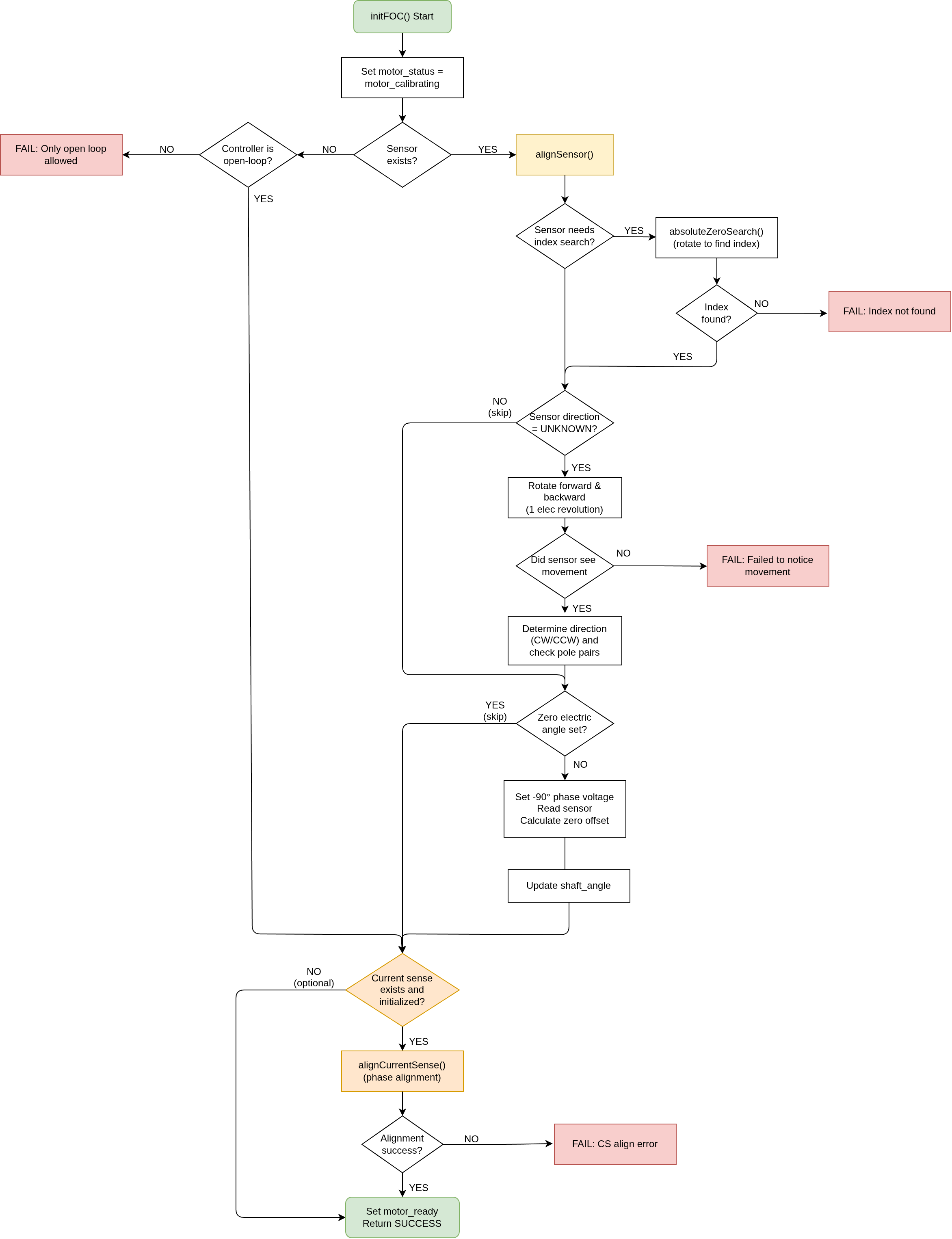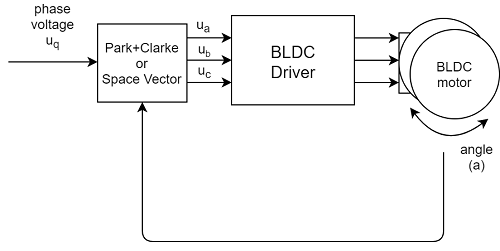# FOC Algorithm implementation v1.6

Since the SimpleFOClibrary is intended for education about the FOC algorithm as well for enabling various applications, there are two (and a half) versions of the FOC modulation implemented in this library. Here I would like to explain you most of the implementation details about the FOC implementation in this library, so that you can better understand what is under the hood and how to change it and adapt for your application.

FOC algorithm has three main components:

• Phase voltage calculation algorithm (modulation): setPhaseVoltage()
• Motor and sensor alignment: initFOC()
• Real-time execution: loopFOC()

Now let’s discuss the implementation details of all three components!

## ❤️ FOC heart function : setting the phase voltage setPhaseVoltage()

SimpleFOClibrary implements two types of FOC PWM modulation. The implemented modulation algorithms are:

• Sinusoidal PWM: SinePWM
• Space Vector PWM: SpaceVectorPWM

You can configure them by setting the value of motor.foc_modulation variable:

motor.foc_modulation = FOCModulationType::SinePWM; // default
// or
motor.foc_modulation = FOCModulationType::SpaceVectorPWM;


NOTE:

The two modulation types and phase voltage calculation is fully implemented in the setPhaseVoltage() function. Here’s how it looks.

void BLDCMotor::setPhaseVoltage(float Uq, float angle_el) {
switch (foc_modulation)
{
case FOCModulationType::SinePWM :
// Sinusoidal PWM modulation
// Inverse Park + Clarke transformation

// angle normalization in between 0 and 2pi
// only necessary if using _sin and _cos - approximation functions
angle_el = normalizeAngle(angle_el + zero_electric_angle);
// Inverse park transform
Ualpha =  -_sin(angle_el) * Uq;  // -sin(angle) * Uq;
Ubeta =  _cos(angle_el) * Uq;    //  cos(angle) * Uq;

// Clarke transform
Ua = Ualpha + voltage_power_supply/2;
Ub = -0.5 * Ualpha  + _SQRT3_2 * Ubeta + voltage_power_supply/2;
Uc = -0.5 * Ualpha - _SQRT3_2 * Ubeta + voltage_power_supply/2;
break;

case FOCModulationType::SpaceVectorPWM :
// Nice video explaining the SpaceVectorModulation (SVPWM) algorithm

// if negative voltages change inverse the phase
// angle + 180degrees
if(Uq < 0) angle_el += _PI;
Uq = abs(Uq);

// angle normalisation in between 0 and 2pi
// only necessary if using _sin and _cos - approximation functions
angle_el = normalizeAngle(angle_el + zero_electric_angle + _PI_2);

// find the sector we are in currently
int sector = floor(angle_el / _PI_3) + 1;
// calculate the duty cycles
float T1 = _SQRT3*_sin(sector*_PI_3 - angle_el) * Uq/voltage_power_supply;
float T2 = _SQRT3*_sin(angle_el - (sector-1.0)*_PI_3) * Uq/voltage_power_supply;
// two versions possible
// centered around voltage_power_supply/2
float T0 = 1 - T1 - T2;
// pulled to 0 - better for low power supply voltage
//float T0 = 0;

// calculate the duty cycles(times)
float Ta,Tb,Tc;
switch(sector){
case 1:
Ta = T1 + T2 + T0/2;
Tb = T2 + T0/2;
Tc = T0/2;
break;
case 2:
Ta = T1 +  T0/2;
Tb = T1 + T2 + T0/2;
Tc = T0/2;
break;
case 3:
Ta = T0/2;
Tb = T1 + T2 + T0/2;
Tc = T2 + T0/2;
break;
case 4:
Ta = T0/2;
Tb = T1+ T0/2;
Tc = T1 + T2 + T0/2;
break;
case 5:
Ta = T2 + T0/2;
Tb = T0/2;
Tc = T1 + T2 + T0/2;
break;
case 6:
Ta = T1 + T2 + T0/2;
Tb = T0/2;
Tc = T1 + T0/2;
break;
default:
// possible error state
Ta = 0;
Tb = 0;
Tc = 0;
}

// calculate the phase voltages and center
Ua = Ta*voltage_power_supply;
Ub = Tb*voltage_power_supply;
Uc = Tc*voltage_power_supply;
break;
}

// set the voltages in hardware
setPwm(Ua, Ub, Uc);
}


## Motor and sensor alignment initFOC()

In order to be able to create the exact 90 degree magnetic field in between stator and rotor, we need to know not just the exact absolute position of the sensor, but also what does that (sensor) position mean for the motor electrical angle. Therefore, before using the FOC algorithm to set the phase voltage we need to align motor electrical angle 0 with sensor angle 0.

The procedure is explained on following diagram.This all happens when we call initFOC() function.

// Function initializing FOC algorithm
// and aligning sensor's and motors' zero position
// - If zero_electric_offset parameter is set the alignment procedure is skipped
//
// - zero_electric_offset  - value of the sensors absolute position electrical offset in respect to motor's electrical 0 position.
// - sensor_direction      - sensor natural direction - default is CW
//
int  BLDCMotor::initFOC( float zero_electric_offset = NOT_SET , Direction sensor_direction = Direction::CW) {
int exit_flag = 1;
// align motor if necessary
// alignment necessary for encoders!
if(zero_electric_offset != NOT_SET){
// absolute zero offset provided - no need to align
zero_electric_angle = zero_electric_offset;
// set the sensor direction - default CW
sensor->natural_direction = sensor_direction;
}else{
// sensor and motor alignment
_delay(500);
exit_flag = alignSensor();
_delay(500);
}

return exit_flag;
}


The initial motor and sensor angle alignment is implemented in the alignSensor() function:

// Encoder alignment to electrical 0 angle
int BLDCMotor::alignSensor() {
if(monitor_port) monitor_port->println("MOT: Align sensor.");

// align the electrical phases of the motor and sensor
float start_angle = shaftAngle();
for (int i = 0; i <=5; i++ ) {
float angle = _3PI_2 + _2PI * i / 6.0;
setPhaseVoltage(voltage_sensor_align,  angle);
_delay(200);
}
float mid_angle = shaftAngle();
for (int i = 5; i >=0; i-- ) {
float angle = _3PI_2 + _2PI * i / 6.0;
setPhaseVoltage(voltage_sensor_align,  angle);
_delay(200);
}
if (mid_angle < start_angle) {
if(monitor_port) monitor_port->println("MOT: natural_direction==CCW");
sensor->natural_direction = Direction::CCW;
} else if (mid_angle == start_angle) {
if(monitor_port) monitor_port->println("MOT: Sensor failed to notice movement");
}

// set angle -90 degrees
// let the motor stabilize for 2 sec
_delay(2000);
// set sensor to zero
sensor->initRelativeZero();
_delay(500);
setPhaseVoltage(0,0);
_delay(200);

// find the index if available
int exit_flag = absoluteZeroAlign();
_delay(500);
if(monitor_port){
if(exit_flag> 0 ) monitor_port->println("MOT: Success!");
else  monitor_port->println("MOT: Not available!");
}
return exit_flag;
}


While the absolute angle alignment is implemented in the function absoluteZeroAlign().

// Encoder alignment the absolute zero angle
// - to the index
int BLDCMotor::absoluteZeroAlign() {

if(monitor_port) monitor_port->println("MOT: Absolute zero align.");
// if no absolute zero return
if(!sensor->hasAbsoluteZero()) return 0;

if(monitor_port && sensor->needsAbsoluteZeroSearch()) monitor_port->println("MOT: Searching...");
// search the absolute zero with small velocity
while(sensor->needsAbsoluteZeroSearch() && shaft_angle < _2PI){
loopFOC();
voltage_q = velocityPID(velocity_index_search - shaftVelocity());
}
voltage_q = 0;
// disable motor
setPhaseVoltage(0,0);

// align absolute zero if it has been found
if(!sensor->needsAbsoluteZeroSearch()){
// align the sensor with the absolute zero
float zero_offset = sensor->initAbsoluteZero();
// remember zero electric angle
zero_electric_angle = normalizeAngle(electricAngle(zero_offset));
}
// return bool if zero found
return !sensor->needsAbsoluteZeroSearch() ? 1 : -1;
}


## Real-time execution loopFOC()

Finally the only thing that is left to do is run the real-time FOC routine. The code needs to get motor position (from sensor), calculate the electrical angle (electricAngle()) from it and set the desired voltage (motor.voltage_q) to the motor phases by using setPhaseVoltage() function.And that is it, this is how it looks in code!

// Function running FOC algorithm in real-time
// it calculates the gets motor angle and sets the appropriate voltages
// to the phase pwm signals
// - the faster you can run it the better Arduino UNO ~1ms, Bluepill ~ 100us
void BLDCMotor::loopFOC() {
// shaft angle
shaft_angle = shaftAngle();
// set the phase voltage - FOC heart function :)
setPhaseVoltage(voltage_q, electricAngle(shaft_angle));
}